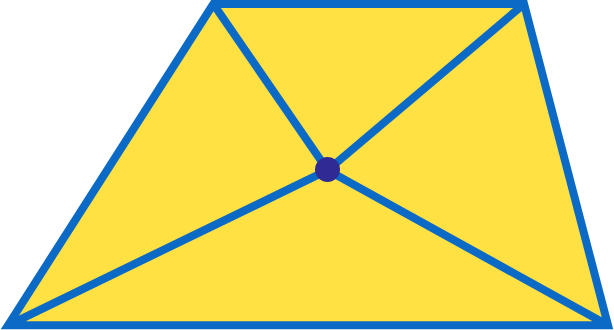# Equally Trapped

Geometry Level 2

Given a trapezoid, is it possible to choose a point in the interior that divides the trapezoid into 4 triangles with equal area by drawing segments from the point to the vertices?Note: For this problem, consider a trapezoid to have exactly one pair of parallel sides.

×